NCERT Exemplar (Part 2): Atoms and Molecules

# NCERT Exemplar (Part 2): Atoms and Molecules - Extra Documents & Tests for Class 9

Q.16. Calcium chloride, when dissolved in water, dissociates into its ions according to the following equation.
CaCl2 (aq) → Ca2+ (aq) + 2CI- (aq)
Calculate the number of ions obtained from CaCl2 when 222 g of it is dissolved in water.
Ans.
CaCl2(aq) → Ca2+(aq) + 2Cl(aq)
1 mole of CaCl2 = 111 g
111 g of CaCl2 = 1 mole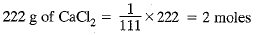1 mole of CaCl2 gives 3 moles of ion
2 moles of CaCl2 gives 6 moles of ion
= 6 x 6.022 x 1023 ions
= 36.132 x 1023 ions = 3.6132 x 1024 ionsQ.17. The difference in the mass of 100 moles each of sodium atoms and sodium ions is 5.48002 g.
Compute the mass of an electron.
Ans.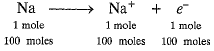100 moles of electrons weigh = 5.48002 g
100 x 6.022 x 1023 electrons weigh = 5.48002 g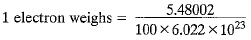= 0.91 x 10-25g = 9.1 x 10-26g = 9.1 x 10-29kg
It is not the real mass of electron.

Q.18. Cinnabar (HgS) is a prominent ore of mercury. How many grams of mercury are present in 225 g of pure HgS? Molar mass of Hg and S are 200.6 g mol-1 and 32 g mol-1 respectively.
Ans.
Molar mass of HgS = 200.6 + 32
= 232.6 g mol-1
232.6 g of HgS contains 200.6 g of pure Hg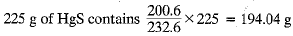Q.19. The mass of one steel screw is 4.11 g. Find the mass of one mole of these steel screws. Compare this value with the mass of the Earth (5.98 x 1024 kg). Which one of the two is heavier and by how many times?
Ans.
Mass of 1 steel screw = 4.11 g
Mass of 6.022 x 1023 steel screws
= 4.11 x 6.022 x 1023 g = 2.475 x 1024 g
= 2.475 x 1021 kg
Therefore, mass of one mole of screws
= 2.475 x 1021 kg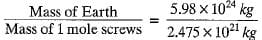= 2.4 x 103
Mass of the earth is 2400 times the mass of 1 mole of screws.

Q.20. A sample of vitamin C is known to contain 2.58 x 1024 oxygen atoms. How many moles of oxygen atoms are present in the sample?
Ans.
Number of moles of oxygen atoms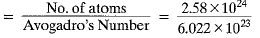= 4.28 moles.

Q.21. Raunak took 5 moles of carbon atoms in a container and Krish also took 5 moles of sodium atoms in another container of the same weight.
(a) Whose container is heavier?
(b) Whose container has more number of atoms?
Ans.
(a) 1 mole of carbon atoms = 12 g
5 moles of carbon atoms = 12 x 5 = 60 g
1 mole of Na = 23 g
5 moles of Na = 23 x 5 = 115 g
Thus, Krish’s container is heavier than that of Raunak.
(b) Both the containers have same number of atoms as both have the same number of moles and both elements are monoatomic.

Q.22. Fill in the missing data in the Table.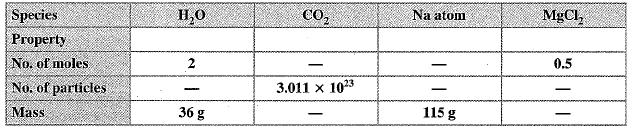Ans.
1 mole of H2O = 1 x 2 + 16 = 18 g
2 moles of H20 = 2 x 18 = 36 g
1 mole of H20 contains 6.022 x 1023 molecule
2 moles of H20 contains 2 x 6.022 x 1023 = 12.044 x 1023 molecules
1 mole of CO2 = 12 + 2 + 16 = 44 g
0.5 mole of CO2 = 44 x 0.5 = 22 g
1 mole of CO2 contains 6.022 x 1023 molecules
0.5 mole of CO2 will contain 0.5 x 6.022 x 1023 = 3.011 x 1023 molecules
1 mole of Na = 23 g
5 moles of Na = 23 x 5 = 115 g
1 mole of Na = 6.022 x 1023 atoms
5 moles of Na = 5 x 6.022 x 1023 = 30.110 x 1023 atoms
1 mole of MgCl2 = 24 + 2 x 35.5 = 24 + 71 = 95 g
0.5 mole of MgCl= 0.5 x 95 = 47.5 g
1 mole of MgCl2 = 6.022 X 1023
0.5 mole of MgCl2 = 0.5 x 6.022 x 1023 = 3.011 x 1023 formula units.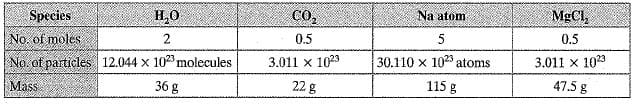Q.23. The visible universe is estimated to contain 1022 stars. How many moles of stars are present in the visible universe?
Ans. Number of moles of stars =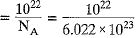= 0.167 x 10-1 = 0.0167 mole.

Q.24. What is the SI prefix for each of the following multiples and sub-multiples of a unit?
(a) 103
(b) 10-1
(c) 10-
(d) 10-6
(e) 10-9
(f) 10-12
Ans.
(a) kilo
(b) deci
(c) centi
(d) micro
(e) nano
(f) pico

25. Express each of the following in kilograms:
(a) 5.84 x 10-3 mg
(b) 58.34 g
(c) 0.584 g
(d) 5.873 x 10-21g
Ans.
(a) 5.84 x 10-3 mg x 10-6 = 5.84 x 10-9 kg [∵ 1 mg = 10-3 g = 10-6 kg]
(b) 58.34 g x 10-3 kg = 5.834 x 10-2 kg [∵ 1 g = 10-3 kg]
(c) 0.584 g = 0.584 x 10-3 kg = 5.84 x 10-4 kg
(d) 5.873 x 10-21 g x 10-3 = 5.873 x 10-24 kg

Q.26. Compute the difference in masses of 103 moles each of magnesium atoms and magnesium ions. (Mass of an electron = 9.1 x 10-31 kg)
Ans.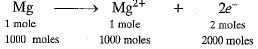1 electron weighs = 9.1 x 10-31 kg
∴ 2000 x 6.022 x 1023 electrons weigh
= 9.1 x 10-31 x 2000 x 6.022 x 1023 kg
= 109.6004 x 10-5 kg = 1.096004 x 10-3 kg

Q.27. Which has more number of atoms? 100 g of N2 or 100 g of NH3
Ans.
1 mole of N2 - 28 g
28 g of N2 = 1 mole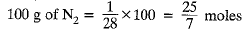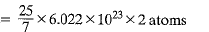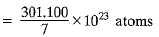= 43.01 x 1023 atoms
= 4.30 x 1024 atoms
1 mole of NH3 = 17 g
17 g of NH3 = 1 mole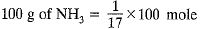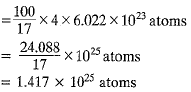Therefore, 100 g of NH3 contains more number of atoms.

Q.28. Compute the number of ions present in 5.85 g of sodium chloride.
Ans.
NaCl → Na+ + Cl-
1 mole of NaCl = 23 + 35.5 = 58.5 g
58.5 g of NaCl = 1 mole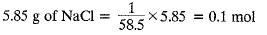1 mole of NaCl gives 2 moles of ions
0.1 mol of NaCl gives 2 x 0.1 = 0.2 mol
= 0.2 x 6.022 x 1023 ions = 1.2044 x 1023 ions

Q.29. A gold sample contains 90% of gold and the rest copper. How many atoms of gold are present in one gram of this sample of gold?
Ans.
Amount of gold in 1 g of 90% pure gold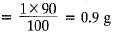1 mole of Au = 197 g1 mole of Au = 6.022 x 1023 atoms
197 g of gold contains 6.022 x 1023 atoms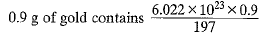= 2.75 x 1021 atoms

Q.30. What are ionic and molecular compounds? Give examples.
Ans.
Ionic compounds are those compounds which are solid and form ions in aqueous solution, have high melting and boiling points, do not conduct electricity in solid state but conduct electricity in molten state or in aqueous solution, e.g. NaCl, KCl, MgO, CaO, etc Molecular compounds may be solids, liquids or gases, do not form ions in aqueous solution, have low melting and boiling points, do not conduct electricity e.g. CH4, CCl4, NH3, PH3, etc.

Q.31. Compute the difference in masses of one mole each of aluminium atoms and one mole of its ions. (Mass of an electron is 9.1 x 10-28 g).
Which one is heavier?
Ans.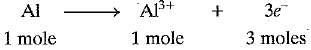Mass of 1 electron = 9.1 x 10-28 g
Mass of 3 x 6.022 x 1023 electrons
= 9.1 x 10-28 x 3 x 6.022 x 1023
Mass of 3 moles of electrons = 164.400 x 10-5 g
= 0.00164 g
Molar mass of Al3+ ions = 27 - 0.00164 g
= 26.9984 g mol-1
Difference in mass between Al and Al3+=0.00164 g
Al atom is heavier than Al3+.

Q.32. A silver ornament of mass ‘m’ gram is polished with gold equivalent to 1% of the mass of silver. Compute the ratio of the number of atoms of gold and silver in the ornament.
Ans.
Mass of silver in the ornament = m gram
Mass of gold in the ornament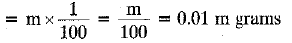108 g of Ag contains 6,022 x 1023 atoms
m gram of Ag contain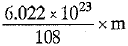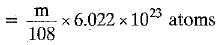197 g of Au contains 6.022 x 1023 atoms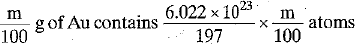Ratio of number of atoms of gold and silver= Au : Ag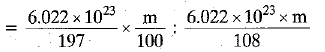= 108 : 19700 = 1 : 182.41
Q.33. A sample of ethane (C2H6) gas has the same mass as 1.5 x 1020 molecules of methane (CH4). How many C2H6 molecules does the sample of gas contain?
Ans.
1 mole of CH4 = 16 g
1 mole of CH4 contains 6.022 x 1023 molecules 6.022 x 1023 molecules of CH4 has mass = 16 g
1.5 x 1020 molecules of CH4 has mass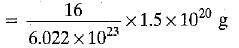Now, 1 mole of C2H6 = 30 g
1 mole of C2H6 = 6.022 x 1023 molecules
30 g of C2H6 contains 6.022 x 1023 moles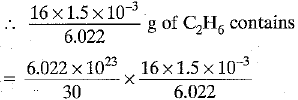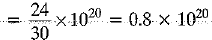= 8 x 1019 molecules

Q.34. Fill in the blanks:
(a) In a chemical reaction, the sum of the masses of the reactants and products remains unchanged. This is called_______.
(b) A group of atoms carrying a fixed charge on them is called_______.
(c) The formula unit m ass of Ca3(PO4)2 is _______ . [Ca = 40 u, P = 31 u, O = 16 u]
(d) Formula of sodium carbonate is _______. and that of ammonium sulphate is______.

Ans. (a) Law of conservation of mass
(c) 40 x 3 + 2 x 31 + 8 x 16
= 120 + 62 + 128 = 310 u
(d) Na2CO3; (NH4)2SO

Q.35. Complete the following crossword puzzle by using the name of the chemical elements. Use the data given in Table.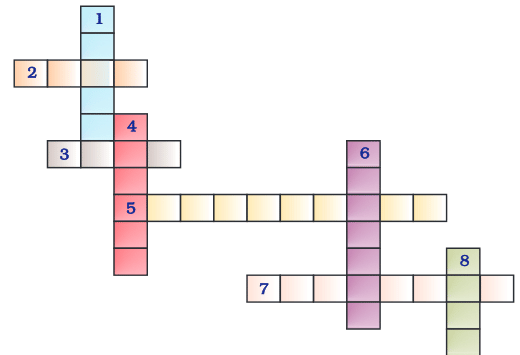Across2. The element used by Rutherford during his a-scattering experiment (4)3. An element which forms rust on exposure to moist air (4)
5. A very reactive non-metal stored under water (10)
7. Zinc metal, when treated with dilute hydrochloric acid, produces a gas of this element which when tested with burning splinter produces a pop sound. (8)
Down
1. A white lustrous metal used for making ornaments and which tends to get tarnished black in the presence of moisture (6)
4. Both brass and bronze are alloys of the element (6)
6. The metal which exists in the liquid state at room temperature (7)
8. An element with symbol Pb (4)
Ans.
> Across :
2. GOLD
3. IRON
5. PHOSPHORUS
7. HYDROGEN
> Down :
1. SILVER
4. COPPER
6. MERCURY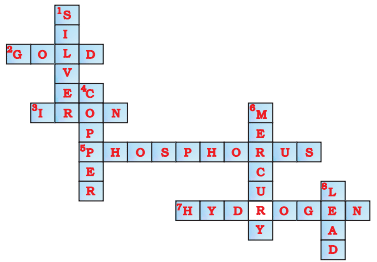Q.36. (a) In the given crossword puzzle, names of 11 elements are hidden. The symbols of these elements are given below. Complete the puzzle.
1. C
2. H
3. Ar
4. O
5. Xe
6. N
7. He
8. F
9. Kr
10. Rn
11. Ne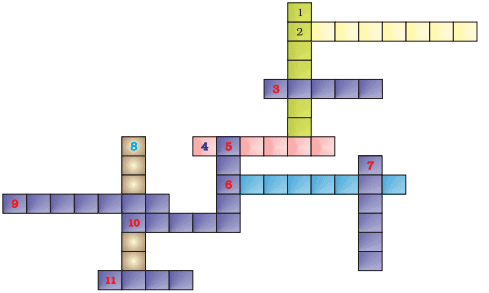(b) Identify the total number of inert gases, their names and symbols from this crossword puzzle.
Ans.
(a)
1. Cl-CHLORINE
2. H-HYDROGEN
3. Ar-ARGON
4. O-OXYGEN
5. Xe-XENON
6. N-NITROGEN
7. He-HELIUM
8. F-FLUORINE
9. Kr-KRYPTON
11. Ne-NEON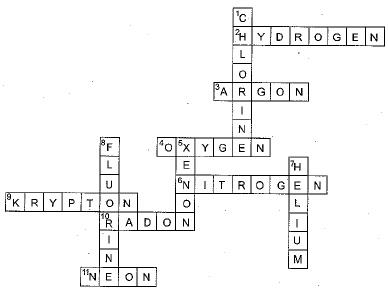(b) Helium (He), Neon (Ne), Argon (Ar), Krypton (Kr), Xenon (Xe) and Radon (Rn) are six inert gases.Q.37. Write the formulae for the following and calculate the molecular mass for each one of them.
(a) Caustic potash
(b) Baking powder
(c) Lime stone
(d) Caustic soda
(e) Ethanol
(f) Common salt
Ans.
(a) Caustic potash is KOH, Molecular mass
= 39 + 16 + 1 = 56 u
(b) Baking powder is NaHC03, Molecular mass
= 23 + 1 + 12 + 48 - 84 u
(c) Lime stone is CaC03, Molecular mass
= 40 + 12 + 48 - 100 u
(d) Caustic soda is NaOH, Molecular mass
= 23 + 16 + 1 = 40 u
(e) Ethanol is C2H5OH, Molecular mass
= 24 + 5 + 16 + 1 = 46 u
(f) Common salt is NaCl, Molecular mass
= 23 + 35.5 = 58.5 u

Q.38. In photosynthesis, 6 molecules of carbon dioxide combine with an equal number of water molecules through a complex series of reactions to give a molecule of glucose having a molecular formula C6H12O6. How many grams of water would be required to produce 18 g of glucose? Compute the volume of water so consumed, assuming the density of water to be 1 g cm-3.
Ans.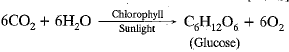Molar mass of C6H12O
= 6 x 12 + 12 x 1 + 6 x 16
= 72 + 12 + 96 = 180 g mol-1
180 g of C6H12O6 needs 108 g of H20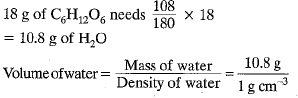Volume of water = 10.8 cm3

The document NCERT Exemplar (Part 2): Atoms and Molecules | Extra Documents & Tests for Class 9 is a part of the Class 9 Course Extra Documents & Tests for Class 9.
All you need of Class 9 at this link: Class 9

## Extra Documents & Tests for Class 9

1 videos|228 docs|21 tests

## FAQs on NCERT Exemplar (Part 2): Atoms and Molecules - Extra Documents & Tests for Class 9

 1. What are atoms and molecules?Ans. Atoms are the smallest units of matter that cannot be divided further. They are made up of protons, neutrons, and electrons. Molecules, on the other hand, are formed when atoms combine chemically. They are composed of two or more atoms held together by chemical bonds.
 2. How is the atomic mass of an element determined?Ans. The atomic mass of an element is determined by taking into account the mass of each isotope of that element and its abundance. The atomic mass is calculated by multiplying the mass of each isotope by its relative abundance, summing up all the products, and expressing the result in atomic mass units (amu).
 3. What is the difference between an element and a compound?Ans. An element is a pure substance that consists of only one type of atom. It cannot be broken down into simpler substances. In contrast, a compound is a substance composed of two or more different elements chemically combined in a fixed ratio. Compounds can be broken down into their constituent elements by chemical means.
 4. How do atoms combine to form molecules?Ans. Atoms combine to form molecules through chemical bonding. There are three types of chemical bonds: covalent, ionic, and metallic. In covalent bonding, atoms share electrons to achieve a stable electron configuration. In ionic bonding, there is a transfer of electrons between atoms, resulting in the formation of ions. Metallic bonding occurs when electrons are delocalized and shared among a lattice of metal atoms.
 5. What is Avogadro's law?Ans. Avogadro's law states that equal volumes of gases, at the same temperature and pressure, contain an equal number of particles (atoms or molecules). This law implies that the number of particles in a gas is directly proportional to its volume. Mathematically, it can be expressed as V ∝ n, where V is the volume and n is the number of particles.

## Extra Documents & Tests for Class 9

1 videos|228 docs|21 testsExplore Courses for Class 9 examSignup to see your scores go up within 7 days! Learn & Practice with 1000+ FREE Notes, Videos & Tests.
10M+ students study on EduRev
Track your progress, build streaks, highlight & save important lessons and more!
Related Searches

,

,

,

,

,

,

,

,

,

,

,

,

,

,

,

,

,

,

,

,

,

;